﻿ Logistic模型在软黏土一维固结水力梯度预测中的应用 Research on Logistic Model of Hydraulic Gradient in One-Dimensional Consolidation of Saturated Clay

Advances in Porous Flow
Vol.08 No.02(2018), Article ID:25326,13 pages
10.12677/APF.2018.82004

Research on Logistic Model of Hydraulic Gradient in One-Dimensional Consolidation of Saturated Clay

Jinzhu Li1, Lu Lan1,2, Xinyu Xie1,2

1Ningbo Institute of Technology, Zhejiang University, Ningbo Zhejiang

2Research Center of Coastal and Urban Geotechnical Engineering, Zhejiang University, Hangzhou Zhejiang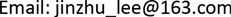Received: May 21st, 2018; accepted: May 31st, 2018; published: Jun. 7th, 2018ABSTRACT

During the consolidation of saturated clay, the drainage rate is positively related to hydraulic gradient. The formula and changeable curve with time of hydraulic gradient are acquired based on Terzaghi’s one-dimensional consolidation, and the “S” type curve is explained in the paper. Logistic function is used instead of complex formula of hydraulic gradient through nonlinear fitting. The relationships between function parameters and soil consolidation parameters are defined to establish Logistic model. One-dimensional consolidation of saturated clay in instantaneous and linear loading conditions is simulated with FEM software, thus comparing changeable curves of hydraulic gradient in two conditions which fits well with Logistic function. The results indicate that Logistic model can not only quantitatively describe variation of hydraulic gradient in Terzaghi’s one-dimensional consolidation, but also other consolidation conditions such as linear loading.

Keywords:Saturated Clay, One-Dimensional Consolidation, Hydraulic Gradient, Logistic Model

Logistic模型在软黏土一维固结水力梯度预测中的应用

1浙江大学宁波理工学院，浙江 宁波

2浙江大学滨海和城市岩土工程研究中心，浙江 杭州Copyright © 2018 by authors and Hans Publishers Inc.

This work is licensed under the Creative Commons Attribution International License (CC BY).

http://creativecommons.org/licenses/by/4.0/1. 引言

2. 水力梯度曲线的一般描述

$i=\frac{2}{{\gamma }_{w}}\frac{p}{H}\sum _{n=0}^{\infty }\mathrm{cos}\frac{Mz}{H}{\text{e}}^{-{M}^{2}{T}_{v}}$ (1)

${T}_{v}=\frac{{c}_{v}t}{{H}^{2}}$ (2)

${c}_{v}=\frac{k{E}_{s}}{{\gamma }_{w}}$ (3)

3. 水力梯度的Logistic模型

3.1. Logistic模型

$i=-\frac{{A}_{1}-{A}_{2}}{1+{\left(t/{t}_{0}\right)}^{\delta }}+{A}_{2}$ (4)

Logistic函数和水力梯度两阶段曲线具备有界性、单调递增性及存在反弯点等共性。公式(4)对t进行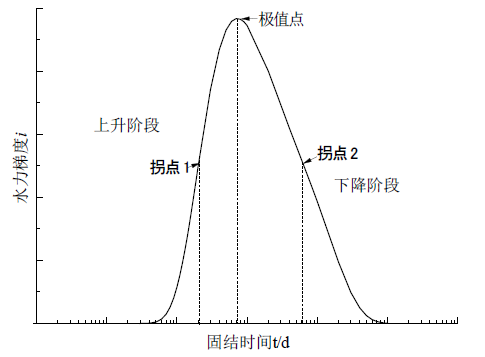Figure 1. Variation of hydraulic gradient with time

${i}^{\prime }=-\frac{\left({A}_{1}-{A}_{2}\right)\delta {\left(t/{t}_{0}\right)}^{\delta -1}}{{\left[1+{\left(t/{t}_{0}\right)}^{\delta }\right]}^{2}{t}_{0}}$ (5)

${i}^{″}=\frac{\left({A}_{1}-{A}_{2}\right)\delta {\left(t/{t}_{0}\right)}^{\delta -2}}{{\left[1+{\left(t/{t}_{0}\right)}^{\delta }\right]}^{3}{t}_{0}{}^{2}}\left[1-\delta +\left(1+\delta \right){\left(\frac{t}{{t}_{0}}\right)}^{\delta }\right]$ (6)

${t}_{1}={\left[1/\left(\lambda -1\right)-1\right]}^{1/\delta }{t}_{0}$ (7)

${t}_{2,3}={\left[\left(\delta -1\right)/\left(\delta +1\right)\right]}^{1/\delta }{t}_{0}$ (8)Figure 2. First derivative of Logistic functionFigure 3. Second derivative of Logistic function

3.2. 算例分析

${i}_{\mathrm{max}}=\frac{\alpha p}{{\gamma }_{w}z}$ (9)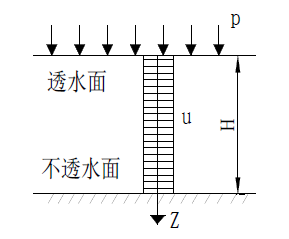Figure 4. Schematic of physical model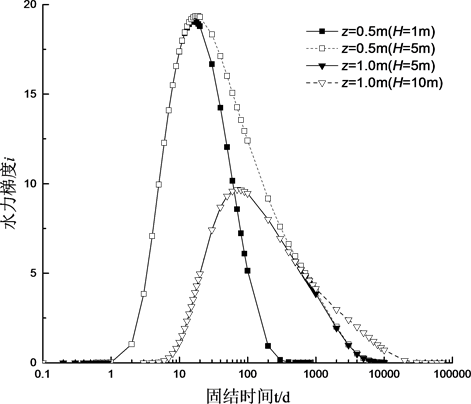Figure 5. Variation of hydraulic gradient i with timeFigure 6. Variation of hydraulic gradient imax with zTable 2. Logistic model and parameters downward

${t}_{0}=\frac{\beta {z}^{2}}{{c}_{v}}$ (10)

${A}_{1}$${A}_{2}$${t}_{0}$$\delta$ 参数值代入式(4)可得上升阶段水力梯度i的Logistic公式，见式(11)。

$i=\frac{\alpha p}{{\gamma }_{w}z}-\frac{\frac{\alpha p}{{\gamma }_{w}z}}{1+{\left(\frac{{c}_{v}t}{\beta {z}^{2}}\right)}^{\delta }}$ (11)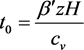(12)

$\delta =A+B\frac{z}{H}$ (13)

$i=\frac{\frac{\alpha p}{{\gamma }_{w}z}}{1+{\left(\frac{{c}_{v}t}{{\beta }^{\prime }zH}\right)}^{A+B\frac{z}{H}}}$ (14)

$i=\frac{\alpha p}{{\gamma }_{w}z}-\frac{\frac{\alpha p}{{\gamma }_{w}z}}{1+{\left(\frac{{c}_{v}t}{\beta {z}^{2}}\right)}^{\delta }},\text{\hspace{0.17em}}\text{\hspace{0.17em}}\text{\hspace{0.17em}}\text{\hspace{0.17em}}\text{\hspace{0.17em}}t<{\left[1/\left(\lambda -1\right)-1\right]}^{1/\delta }{t}_{0}$ (15)

$i=\frac{\frac{\alpha p}{{\gamma }_{w}z}}{1+{\left(\frac{{c}_{v}t}{{\beta }^{\prime }zH}\right)}^{A+B\frac{z}{H}}},\text{\hspace{0.17em}}\text{\hspace{0.17em}}\text{\hspace{0.17em}}\text{\hspace{0.17em}}\text{\hspace{0.17em}}t\ge {\left[1/\left(\lambda -1\right)-1\right]}^{1/\delta }{t}_{0}$ (16)

3.3. 数值模拟

3.3.1. 瞬时加载结果分析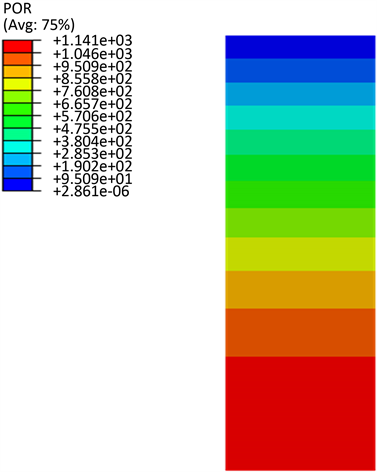Figure 7. Distribution of pore pressure (t = 10,000 d)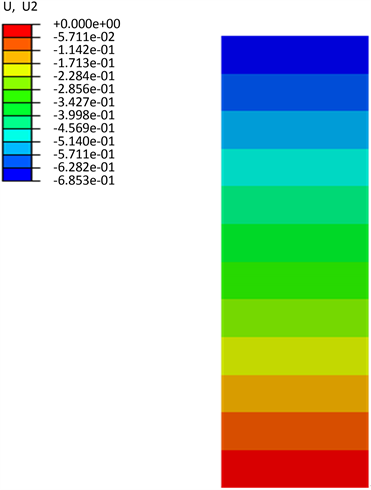Figure 8. Distribution of settlement (t = 10,000 d)Figure 9. Distribution of settlement (t = 10,000 d)Figure 10. Distribution of settlement (t = 10,000 d)

$i=\left\{\begin{array}{l}6.6-\frac{6.64}{1+{\left(t/45\right)}^{2.84}},\text{\hspace{0.17em}}\text{\hspace{0.17em}}\text{\hspace{0.17em}}\text{\hspace{0.17em}}\text{\hspace{0.17em}}\text{\hspace{0.17em}}t\le 153\text{\hspace{0.17em}}\text{d}\\ \frac{6.64}{1+{\left(t/984.375\right)}^{1.696}},\text{\hspace{0.17em}}\text{\hspace{0.17em}}\text{\hspace{0.17em}}\text{\hspace{0.17em}}\text{\hspace{0.17em}}t>153\text{\hspace{0.17em}}\text{d}\end{array}$ (17)

3.3.2. 线性加载结果分析

$i=\left\{\begin{array}{l}6.51-\frac{6.51}{1+{\left(t/62\right)}^{3.49}},\text{\hspace{0.17em}}\text{\hspace{0.17em}}\text{\hspace{0.17em}}\text{\hspace{0.17em}}t\le 189\text{\hspace{0.17em}}\text{d}\\ \frac{6.51}{1+{\left(t/1160\right)}^{1.75}},\text{\hspace{0.17em}}\text{\hspace{0.17em}}\text{\hspace{0.17em}}\text{\hspace{0.17em}}\text{\hspace{0.17em}}\text{\hspace{0.17em}}\text{\hspace{0.17em}}\text{\hspace{0.17em}}\text{\hspace{0.17em}}\text{\hspace{0.17em}}t>189\text{\hspace{0.17em}}\text{d}\end{array}$ (18)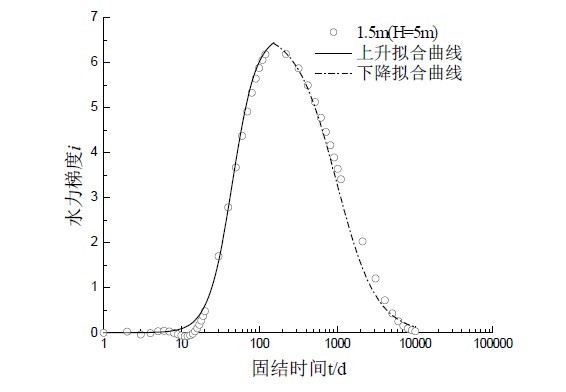Figure 11. Comparison of ABAQUS numerical solution and formula (15)Figure 12. Variation of linear load with time

4. 结论

1) 水力梯度在固结过程中随时间先增大后减小，其变化曲线分为上升和下降两阶段的S型曲线，且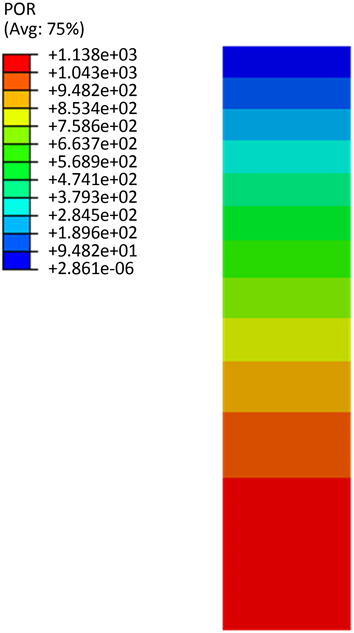Figure 13. Distribution of pore pressure after consolidationFigure 14. i-t curve of ABAQUS numerical solution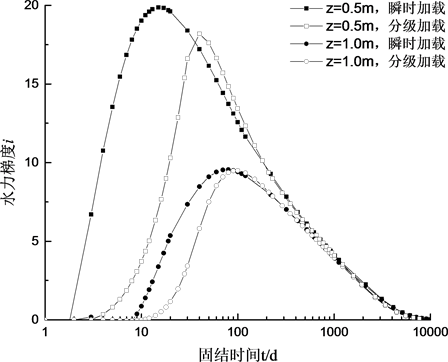Figure 15. i-t curve of instantaneous load and linear load

2) 水力梯度的Logistic模型中，无量纲参数α、β等取值应视具体情况考虑。本文以Terzaghi一维固结解析解为基础，虽存在一定的拟合误差，但能较好反映固结过程中水力梯度的变化规律，预测固结排水速率，分析固结过程机理。

Research on Logistic Model of Hydraulic Gradient in One-Dimensional Consolidation of Saturated Clay[J]. 渗流力学进展, 2018, 08(02): 22-34. https://doi.org/10.12677/APF.2018.82004

1. 1. 代革联. 对水力梯度的理解及其应用分析[J]. 西北地质, 1997, 18(4): 102-105.

2. 2. 陈建生, 李兴文. 堤防管涌产生集中渗漏通道机理与探测方法研究[J]. 水利学报, 2000, 9(9): 48-54.

3. 3. 周健, 姚志雄. 管涌发生发展过程的细观试验研究[J]. 地下空间与工程学报, 2007, 3(5): 842-848.

4. 4. 房后国, 刘娉慧. 海积软土排水固结机理分析[J]. 吉林大学学报: 地球科学版, 2005, 35(2): 207-212.

5. 5. S. Hansbo. Consolidation equation valid for both Darcian and non-Darcianflow[J]. Géotechnique, 2001, 51(1):51-54. https://doi.org/10.1680/geot.2001.51.1.51

6. 6. 齐添, 谢康和. 萧山粘土非达西渗流性状的试验研究[J]. 浙江大学学报, 2007, 41(6): 1023-1028.

7. 7. 冯晓腊. 饱和粘性土的渗透固结特性及其微观机制的研究[J]. 水文地质工程地质, 1991, 18(1): 6-11.

8. 8. 王秀艳. 对粘性土孔隙水渗流规律本质的新认识[J]. 地球学报, 2003, 24(1): 91-95.

9. 9. Liu, H.H. and Jens, B. (2012) On the Relationship between Water Flux and Hydraulic Gradient for Unsaturated and Saturated Clay. Journal of Hydrology, 475, 242-247. https://doi.org/10.1016/j.jhydrol.2012.09.057

10. 10. Griffiths, F.J. and Joshi, R.C. (1989) Change in Pore Size Distribution Due to Consolidation of Clays. Geotechnique, 39, 159-167. https://doi.org/10.1680/geot.1989.39.1.159

11. 11. Fenton, G.A. and Griffiths, D.V. (1997) Extreme Hydraulic Gradient Statistics in Stochastic Earth Dam. Journal of Geotechnical and Geoenvironmental Engineering, 123, 995-1000. https://doi.org/10.1061/(ASCE)1090-0241(1997)123:11(995)

12. 12. 黄杰卿. 非线性大应变固结理论与试验研究[D]: [博士学位论文]. 杭州: 浙江大学, 2015.

13. 13. 吴健. 饱和软土复杂非线性大变形固结特性及应用研究[D]: [博士学位论文]. 杭州: 浙江大学, 2008.

14. 14. 杨少丽, 齐剑峰. 桶基负压沉贯下粉土中水力梯度的变化过程[J]. 岩土工程学报, 2004, 25(6): 662-665.

15. 15. 宰金珉, 梅国雄. 成长曲线在地基沉降预测中的应用[J]. 南京建筑工程学院学报(自然科学版), 2000(2): 8-13.

16. 16. 梅国雄, 宰金珉. 沉降时间曲线呈“S”型的证明——从一维固结理论角度[J]. 岩土力学, 2004, 25(1): 20-22.